#### Vol. 63, No. 1, 1976

 Download this articleFor screen For printingRecent Issues Vol. 325: 1 Vol. 324: 1  2 Vol. 323: 1  2 Vol. 322: 1  2 Vol. 321: 1  2 Vol. 320: 1  2 Vol. 319: 1  2 Vol. 318: 1  2Online Archive Volume: Issue:The Journal Subscriptions Editorial Board Officers Contacts Submission Guidelines Submission Form Policies for Authors ISSN: 1945-5844 (e-only) ISSN: 0030-8730 (print) Special Issues Author Index To Appear Other MSP Journals
Fundamental units and cycles in the period of real quadratic number fields. I

### Leon Bernstein

Vol. 63 (1976), No. 1, 37–61
##### Abstract

0. Introduction. In this paper we introduce the concept of “Cycles in the Period” of the simple continued fraction expansion of a real quadratic irrational. This is expressed in the

Definition. Let M, D, d be positive rational integers, M square free, M = D2 + d, d 2D. Let k, a, s be nonnegative rational integers, 0 a k 1; let f = f(k,a,s;d,D) be a polynomial with rational integral coefficients. For a fixed s, the finite sequence of polynomials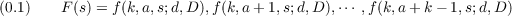will be called “Cycle in the Period” of the simple continued fraction expansion of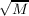if, for s0 1, this expansion has the form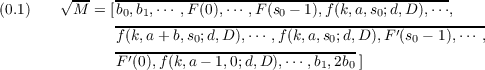b 1;b k 1;k is the length of the cycle; F(s) means that the order of the f s must be reversed.

In the first part of this paper, the main result is the construction of infinitely many classes of quadratic fields Q(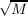), each containing infinitely many M of a simple structure. Among the various classes thus constructed, there are a few in whose expansion of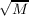cycles in the period surprisingly have the length 12. Functions f(k,a,s;d,D), f(k,a + 1,s;d,D), are of course stated explicitly; hence we are able to construct numbers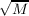such that the primitive period of their expansion has any given length m which is a function of the parameter k.

##### Mathematical Subject Classification
Primary: 12A45, 12A45
Secondary: 10A30
##### Milestones
Published: 1 March 1976
##### Authors
 Leon Bernstein